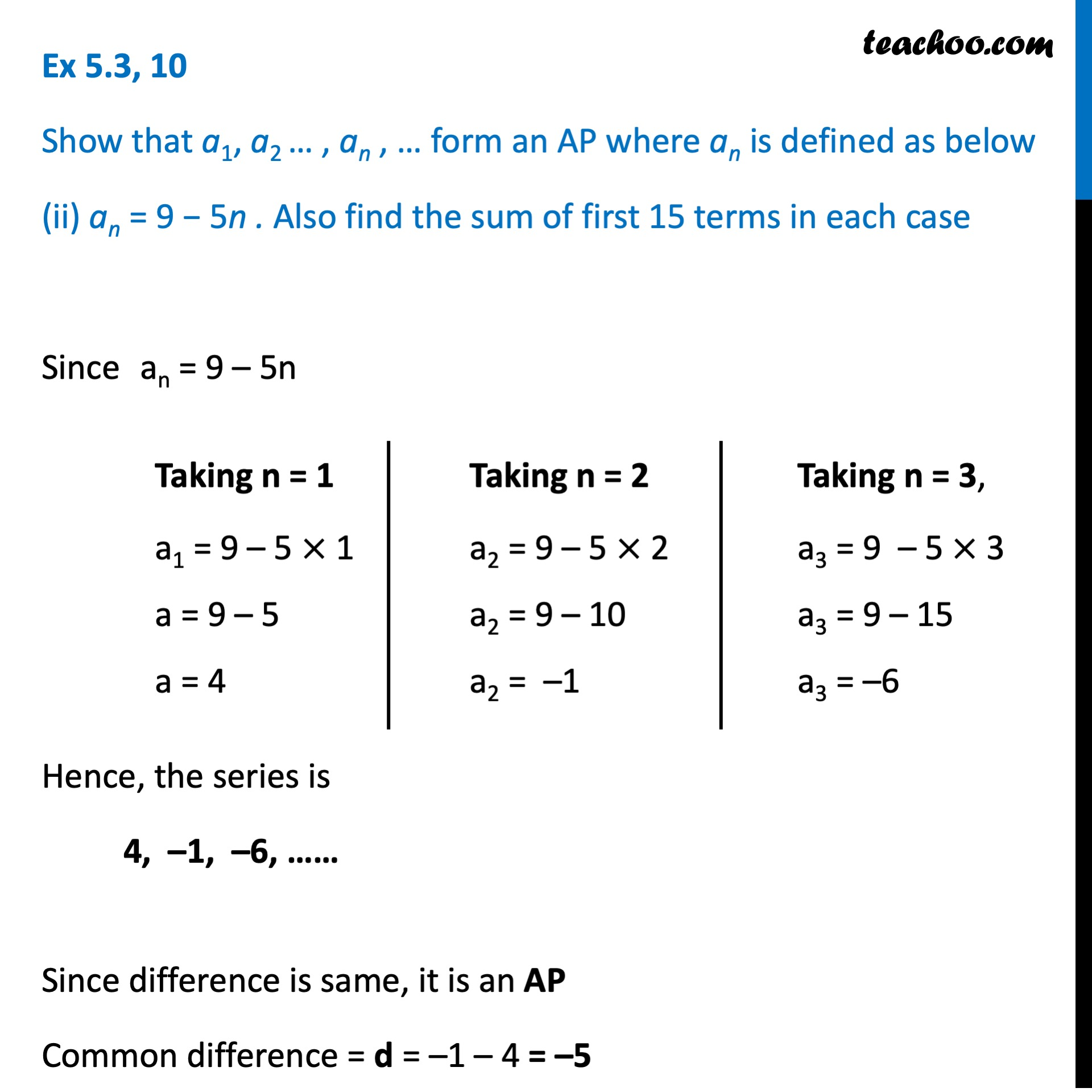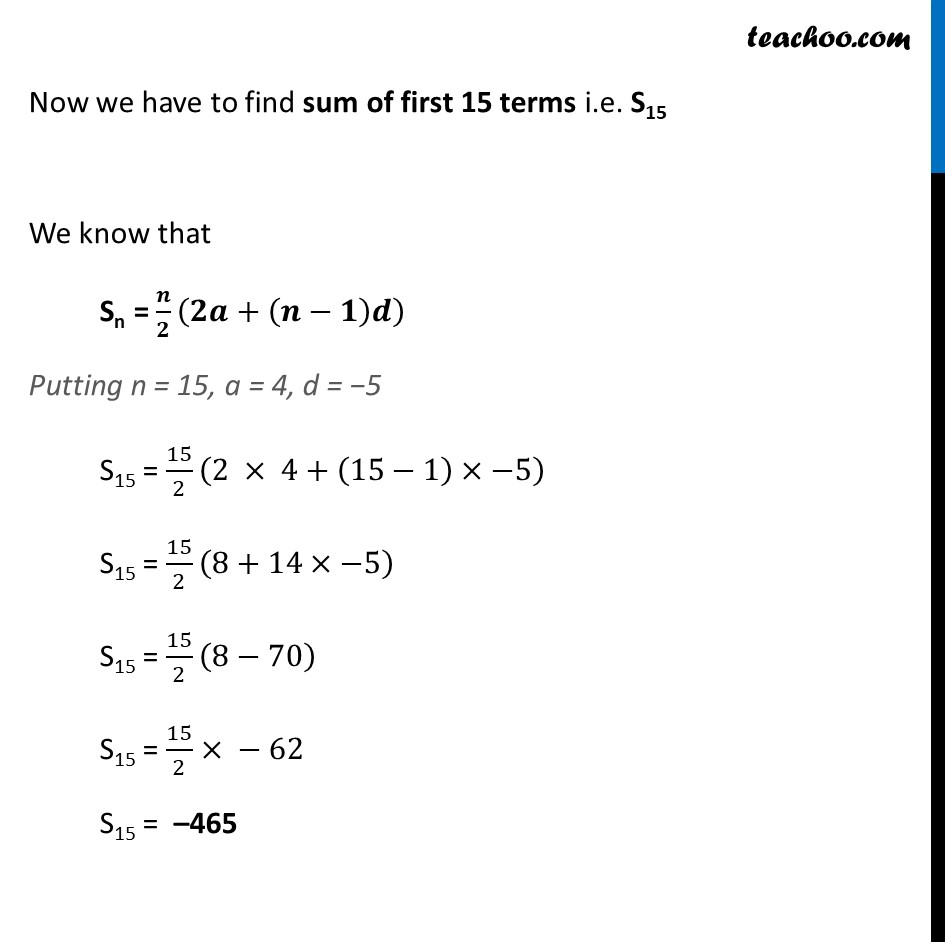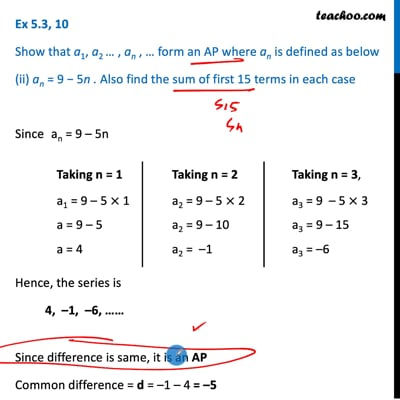Ex 5.3

Chapter 5 Class 10 Arithmetic Progressions (Term 2)
Serial order wiseThis video is only available for Teachoo black users

### Transcript

Ex 5.3, 10 Show that a1, a2 … , an , … form an AP where an is defined as below (ii) an = 9 − 5n . Also find the sum of first 15 terms in each case Since an = 9 – 5n Hence, the series is 4, –1, –6, …… Since difference is same, it is an AP Common difference = d = –1 – 4 = –5 Taking n = 1 a1 = 9 – 5 × 1 a = 9 – 5 a = 4 Taking n = 2 a2 = 9 – 5 × 2 a2 = 9 – 10 a2 = –1 Taking n = 3, a3 = 9 – 5 × 3 a3 = 9 – 15 a3 = –6 Now we have to find sum of first 15 terms i.e. S15 We know that Sn = 𝒏/𝟐 (𝟐𝒂+(𝒏−𝟏)𝒅) Putting n = 15, a = 4, d = −5 S15 = 15/2 (2 × 4+(15−1)×−5) S15 = 15/2 (8+14×−5) S15 = 15/2 (8−70) S15 = 15/2× − 62 S15 = –465